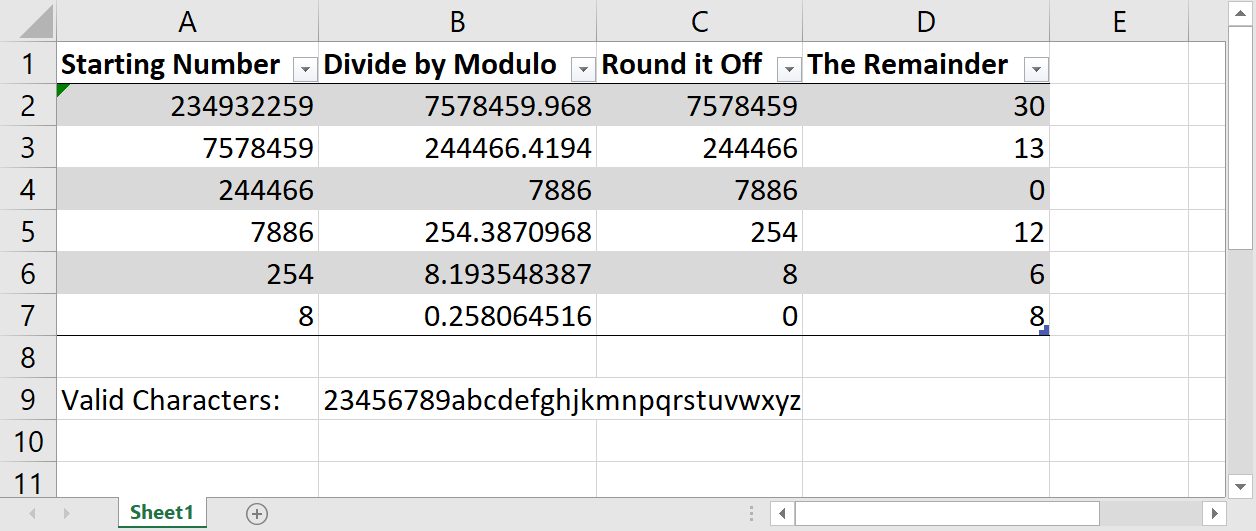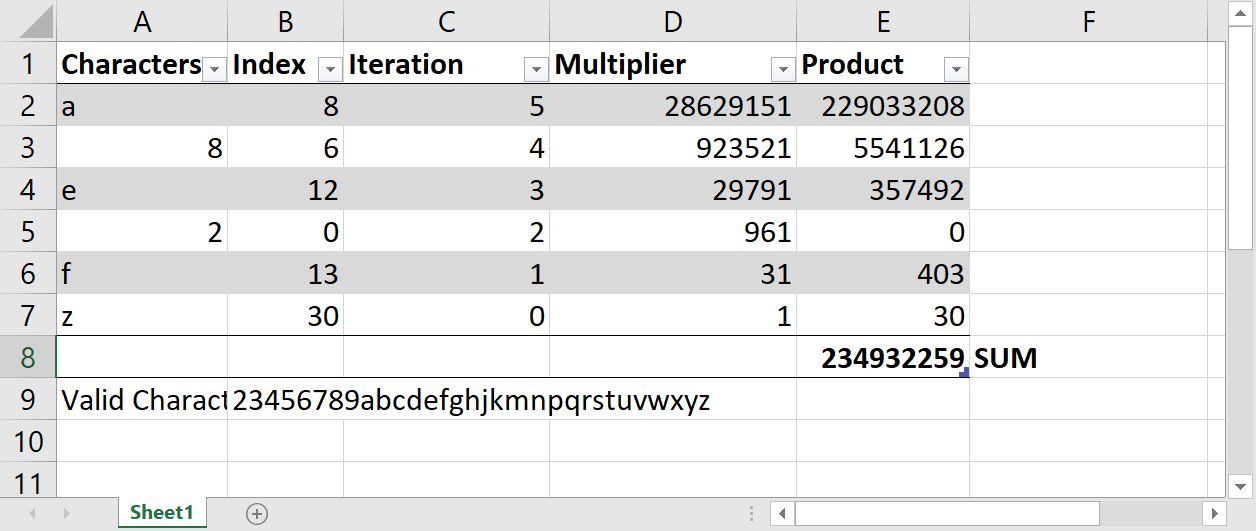ngjackson

# Generating Alphanumeric Sequential IDs in Java

I came across an interesting situation in work the other day while working on an internal project (a link shortener, similar to bitly). I wanted to generate a unique, sequential ID that was as short as possible, but also easy to read and type. This meant using letters as well as numbers, but don't use any homoglyphs like O and 0, or l and 1.

After working it out a bit with a coworker, we came up with a final solution which can be found in this gist. I'll be stepping through a Java implementation, but this should be doable in any language.

## Getting IDs from Numbers

Let's start by getting IDs from numbers, arguably the more simple and straight forward task. What we need to do is convert a number to an alphanumeric string that only uses a defined set of characters. To do this, we need some math.

In order to represent numbers in the least number of characters possible, we need to be able to use more characters than the standard numbers `0-9`. In this case, we'll use letters (just lower case, to keep things simple, but you could include upper case, special characters, even emojis if you wanted to!). Here are the characters we'll be working with:

``23456789abcdefghjkmnpqrstuvwxyz``

Notice I've used 0-9, a-z, but I've taken out the most common homoglyphs.

So how do we go from a number like `234932259` to something like `a8e2fz`? It all has to do with creating a base-n number system. (The rest of this post assumes you know how binary works. If not, check out this quick guide.) Instead of using binary (which is base 2), or decimal (which is base 10), we need a custom base. Binary is base 2 because you have 2 possible digits: 0 and 1. Decimal is base 10 because you have 10 possible digits: 0-9. We have 31 available characters to chose from (given the list above), so our system is going to be base 31. 31 is a special number for the purposes of this post.

This table nicely summarizes the flow from beginning to end:The starting number is placed in `A2`. From there, we take the number and divide it by the length of our valid characters, in this case, 31. If we take off the remainder, this will give us the starting point for the next division. But before we go onto the next division, we need to find what the remainder is. The `=MOD()` function gives us that information exactly.

What we've learned so far is this: `31^1` will go into 234932259 7578459 times with a remainder of 30. This doesn't seem like much, but we will see how this is used in the next step.

Now, instead of starting with 234932259 , we want to start with the whole number from the previous step. Why? We already know how many times `31^1` goes into 234932259 , now we need to find out how many times `31^2` goes into 234932259 . And since dividing by 31 twice is the same as dividing by `31^2`, we can just use the whole number from the previous step, and that's what we do on row 3. We start with 7578459 and repeat the process over again, this time we get a remainder of 13.

We continue the process until the starting number is 0. At that point, 31 will not divide into it anymore.

So now, we have this list of remainders: 30, 13, 0, 12, 6, and 8. What do we do with them? Well, let's think what they mean. Based on our calculations, we know the following is true:

``````Divisor   | 31^0 | 31^1 | 31^2 | 31^3 | 31^4 | 31^5
---------------------------------------------------
Remainder |  30  |  13  |  0   |  12  |  6   |  8  ``````

This is the exact data we need to create our base-31 number, but it's actually backwards. Remember, numbers are read from right to left in increasing order, so we need to switch the order. Now we have this list of numbers: `8, 6, 12, 0, 13, 30`.

The final step is simply to convert the numbers into alphanumeric characters, and we're done! We first find the 8th character in our list of valid characters, then the 13th character, and the 0th character, etc. until the end, and push all the characters together and we get `a8e2fz`, our final product.

### Java Implementation

This is more of a logic exercise than it is a Java exercise, so once you know the logic, the code is pretty straight forward.

Here's how I wrote it:

``````/**
* Go from number (like 12345) to alphanumeric ID (like a8e7z4)
*
* @param number The number you wish to convert to ID
* @return the alphanumeric ID as a string
*/

public static String getIdFromNumber(Integer number) {
// The magic number we're trying to get down to 0
long magicNumber = number;

// Some placeholder values
long wholeNum = 0;
int remainder = 0;
String id = "";

// The value we'll use as our modulo, just stored in a variable because it's simpler
int modVal = VALID_CHARACTERS.length();

// Loop until the magic number (AKA the whole number) is not zero (>0 also works)
while (magicNumber != 0) {

// First, take the magic number, divide it by the modulo value, and store that as our whole
// number
wholeNum = (long) Math.floor(magicNumber / modVal);

// Next, find the remainder of when we take the magic number and divide it by the modulo value
remainder = (int) magicNumber % modVal;

// Then, take the remainder that we just got, find what position it is in our valid
// characters, and store it in our ID (yes, this means the ID is backwards, but we fix that
// later)
id += VALID_CHARACTERS.charAt(remainder);

// Lastly, make the magic number the whole number from above (since we don't need to deal with
// the remainder)
magicNumber = wholeNum;

// Loop again until the whole num is zero!

}

// One last thing: reverse the string. StringBuilder has a nice reverse method, so that's what
// I'm using to reverse the string.
return new StringBuilder(id).reverse().toString();

}
``````

The only slightly strange thing about this code is that there's no great way to reverse a string in Java, surprisingly, which is why I had to reach for the `StringBuilder` which does have a nice method.

You'll also notice that I convert the remainder to the character as I go and then reverse the string, instead of reversing then finding the index as I explained in my logic above.

## Getting Numbers from IDs

Now that we know roughly how our base-31 number system works, getting numbers from the IDs is pretty straight forward.

Here's another table that shows an overview of the steps:It's easier to read/understand if you start at the bottom and work your way up. Starting with the last character, `z`, we first get its index in the array of valid characters, which is 30. The index becomes what we multiply the multiplier by (probably could have chosen better names for those). The multiplier is the result of our base (31) to the power of the "position" in our string. In this case, it's the first letter we've looked at, so it's at position zero. We get the product, and set it aside for the time being.

Next, we look at the character `f`. Its index in the array is 13. The multiplier for this character is the result of our base (31 still) to the power of the position, which is 1 (one more than last time). Work it all out, get the product, and move on to the next one!

Once we've followed these steps for all of the characters, add up all of the products and we get our final number in base 10.

### Java Implementation

Again, the complexity is in the logic, not the Java implementation. All of the heavy lifting is done in one line for this function.

Here's how I implemented it:

``````/**
* Go from alphanumeric ID (like a8e7z4) to number (like 12345)
*
* @param id The string with the alphanumeric ID
* @return The number as an int
*/

public static Integer getNumberFromId(String id) {

// A placeholder for the base 10 number
Integer base10Number = 0;

// Loop over each character in the input string
for (int i = 0; i < id.length(); i++) {

// Get the "current" character
char c = id.charAt(i);

// Find where that character is in the list of valid characters
int index = VALID_CHARACTERS.indexOf(c);

// Check if the character was able to be found
if (index == -1) {
throw new IllegalArgumentException("Character \"" + c
+ "\" is not a valid character. The only valid characters are: " + VALID_CHARACTERS);
}

// Take the base (31) to the power of the total number of characters, minus i, minus 1, and
// mutiply it by the index
base10Number += (int) Math.pow(VALID_CHARACTERS.length(), id.length() - i - 1) * index;

}

return base10Number;

}
``````

The only edge case we have to take care of is if someone passes in a character in the ID that's not in our array, a 1 or a 0 for example. In that case, I just throw an exception.

## Summary

This was a fun, challenging algorithm to write. We take a number and turn it into a new alphanumeric ID based upon a list of valid characters. The best part is that because of how the algorithm is written, you can change up the `VALID_CHARACTERS` string (to add uppercase characters, for example) and not have to change any other code!

Let me know if you have any thoughts or feedback in the comments below!